# The PHREG Procedure

### Assessment of the Proportional Hazards Model

Subsections:

The proportional hazards model specifies that the hazard function for the failure time T associated with a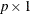column covariate vectortakes the form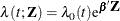where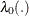is an unspecified baseline hazard function andis acolumn vector of regression parameters. Lin, Wei, and Ying (1993) present graphical and numerical methods for model assessment based on the cumulative sums of martingale residuals and their transforms over certain coordinates (such as covariate values or follow-up times). The distributions of these stochastic processes under the assumed model can be approximated by the distributions of certain zero-mean Gaussian processes whose realizations can be generated by simulation. Each observed residual pattern can then be compared, both graphically and numerically, with a number of realizations from the null distribution. Such comparisons enable you to assess objectively whether the observed residual pattern reflects anything beyond random fluctuation. These procedures are useful in determining appropriate functional forms of covariates and assessing the proportional hazards assumption. You use the ASSESS statement to carry out these model-checking procedures.

For a sample of n subjects, let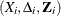be the data of the ith subject; that is,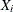represents the observed failure time,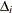has a value of 1 ifis an uncensored time and 0 otherwise, and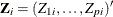is a p-vector of covariates. Let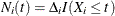and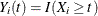. Let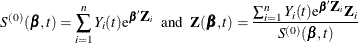Let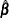be the maximum partial likelihood estimate of, and let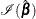be the observed information matrix.

The martingale residuals are defined as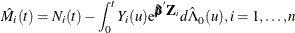where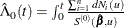.

The empirical score process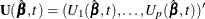is a transform of the martingale residuals: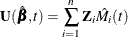#### Checking the Functional Form of a Covariate

To check the functional form of the jth covariate, consider the partial-sum process of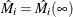: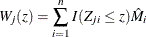Under that null hypothesis that the model holds,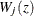can be approximated by the zero-mean Gaussian process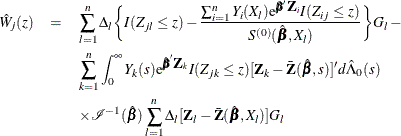where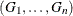are independent standard normal variables that are independent of,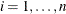.

You can assess the functional form of the jth covariate by plotting a small number of realizations (the default is 20) of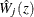on the same graph as the observedand visually comparing them to see how typical the observed pattern ofis of the null distribution samples. You can supplement the graphical inspection method with a Kolmogorov-type supremum test. Let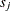be the observed value of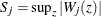and let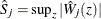. The p-value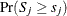is approximated by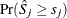, which in turn is approximated by generating a large number of realizations (1000 is the default) of.

#### Checking the Proportional Hazards Assumption

Consider the standardized empirical score process for the jth component of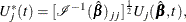Under the null hypothesis that the model holds,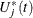can be approximated by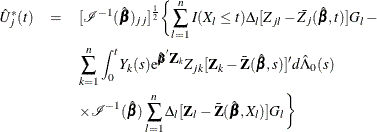where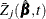is the jth component of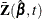, andare independent standard normal variables that are independent of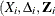,.

You can assess the proportional hazards assumption for the jth covariate by plotting a few realizations of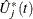on the same graph as the observedand visually comparing them to see how typical the observed pattern ofis of the null distribution samples. Again you can supplement the graphical inspection method with a Kolmogorov-type supremum test. Let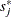be the observed value of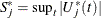and let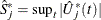. The p-value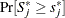is approximated by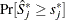, which in turn is approximated by generating a large number of realizations (1000 is the default) of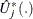.